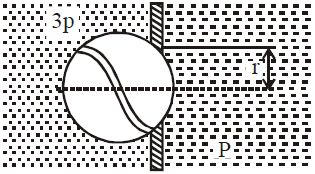# Force on Ball!A ball covers the hole of radius $r$ in a plane vertical wall (figure). The wall separates left side and right side which have different fluid pressure $3p$ and $p$. With what force does the liquid press the ball to the hole? If force is $np \pi r^2$, find $n$.

×